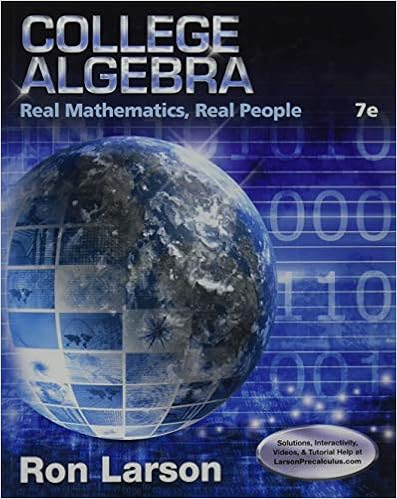# Find the particular solution y f x to the given

• Notes
• 5
• 50% (2) 1 out of 2 people found this document helpful

This preview shows page 4 - 5 out of 5 pages.

##### We have textbook solutions for you!
The document you are viewing contains questions related to this textbook.The document you are viewing contains questions related to this textbook.
Chapter 3 / Exercise 3
College Algebra: Real Mathematics, Real People
LarsonExpert Verified
Find the particular solution ( )yfx=to the given differential equation with the initial condition f0( )=3.
##### We have textbook solutions for you!
The document you are viewing contains questions related to this textbook.The document you are viewing contains questions related to this textbook.
Chapter 3 / Exercise 3
College Algebra: Real Mathematics, Real People
LarsonExpert Verified
12.(1999, AB-4) Suppose that the function fhas a continuous second derivative for all x, and that ( )02f( )03f=, and ( )00f′′=. Let gbe a function whose derivative is given by ( )( )( )()232xgxefxfx=+for all x(a)Write an equation of the tangent line to the graph of fat the point where 0x=(b)Is there sufficient information to determine whether or not the graph of fhas a point of inflection when 0x=? Explain your answer. (c)Given that ( )04g=, write an equation of the line tangent to the graph of gat the point where 0x(d)Show that ( )( )( )( )()262xgxefxfxfx′′′′=+. Does ghave a local maximum at 0x=? Justify your answer. =, . . =.
•••